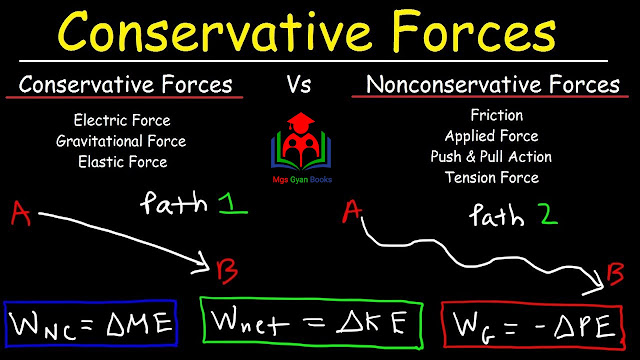# Conservative and Non-conservative forces संरक्षित और असंरक्षित बल

### What do you mean by Conservative and Non-conservative forces?

#### Conservative and Non-conservative forces#### Conservative Force:

A  force is said to be conservative, if the amount of work done in moving any object against that the force is independent of how the object moves from initial  to the final position.
Example: Gravitational force, force exerted by a spring, electostatic force between two charge ect.

#### Non-conservative Force:

A  force is said to be non-conservative, if the amount of work done by that force on an object moving between two points depends on the path taken between the points and the work does not zero
Example: Force of friction this an example of non-conservative forces.

दोस्तों अगर आप चाहते हैं की मैं इसके आगे का  Prove that the work done by conservative force in a round trip is zero.
पोस्ट करूं तो मुझे कमेंट करके जरूर  बताएं और अपने दोस्तों को भी जरूर शेयर करें।